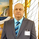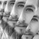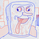3957 vues
This is an experimental study which calculates a linear regression channel over a specified period or interval using custom moving average types for its calculations.

Linear regression is a linear approach to modeling the relationship between a dependent variable and one or more independent variables.
In linear regression , the relationships are modeled using linear predictor functions whose unknown model parameters are estimated from the data.

The regression channel in this study is modeled using the least squares approach with four base average types to choose from:
-> Arnaud Legoux Moving Average ( ALMA )
-> Exponential Moving Average ( EMA )
-> Simple Moving Average ( SMA )
-> Volume Weighted Moving Average ( VWMA )
When using VWMA , if no volume is present, the calculation will automatically switch to tick volume , making it compatible with any cryptocurrency, stock, currency pair, or index you want to analyze.

There are two window types for calculation in this script as well:
-> Continuous, which generates a regression model over a fixed number of bars continuously.
-> Interval, which generates a regression model that only moves its starting point when a new interval starts. The number of bars for calculation cumulatively increases until the end of the interval.

The channel is generated by calculating standard deviation multiplied by the channel width coefficient, adding it to and subtracting it from the regression line, then dividing it into quartiles.

To observe the path of the regression, I've included a tracer line, which follows the current point of the regression line. This is also referred to as a Least Squares Moving Average ( LSMA ).

For added predictive capability, there is an option to extend the channel lines into the future.

A custom bar color scheme based on channel direction and price proximity to the current regression value is included.

I don't necessarily recommend using this tool as a standalone, but rather as a supplement to your analysis systems.
Regression analysis is far from an exact science. However, with the right combination of tools and strategies in place, it can greatly enhance your analysis and trading.
Feb 16
-> Script structure has been reorganized.
-> Corrected NaN filter values in initial states.
-> Added Rolling Moving Average and Linear Weighted Moving Average to the list of available base averages.
-> Added style customization for the channel lines. Because TV doesn't have built-in style inputs for line objects, you'll find these settings in the "Inputs" section. For each channel line, you can now adjust:
- If the line is drawn - selected via the specified toggle switch
- Width of the line - can be any integer greater than zero
- Style of the line - available styles are solid, dotted, and dashed
- Color of the line - uses my RGB color editor, which has 216 colors to choose from via the specified R, G, and B inputs

## CommentairesWhat three parameters we are expecting here?

//Correlation Coefficient
corr(x, y, t)=>

Close, length and something else?

Thanks.
Répondresyracusepro
@syracusepro, Great question. x is the bar number counting upward, y is the price value, and t is the sampling length. The slope parameter b is dependent on correlation between price and time.
RépondreThank you for the new script once again. I'm a big fan!
RépondreI'm a big fan. Thank you for the great indicator.

I have one thing to wanna know. Please let me know the value of the Least Squares Moving Average which is a magic line for me: with which I set each lsma of each minute-hour-day-week-month-chart in a lump sum IN MY Every Chart from 1m through 15m/1h/2h/4h/12h charts to even 1D and 3D and 1W/1M charts.

In short, how can I see each chart's LSMA in one chart: with which I can see the various charts' LSMA lines of flow in ONE chart - I mean, I can see all charts' lsma lines in one chart even though which minute-chart it is.
Répondre